New offer! Get 30% off one whole year of Unlimited learning. Subscribe for just £249.99 £174.99. New subscribers only T&Cs apply

•# Merge() parameters

Merge () parameters

Now, let’s explore some examples using various parameters in the merge function.

## Example 1: Inner joins

Look at these dataframes: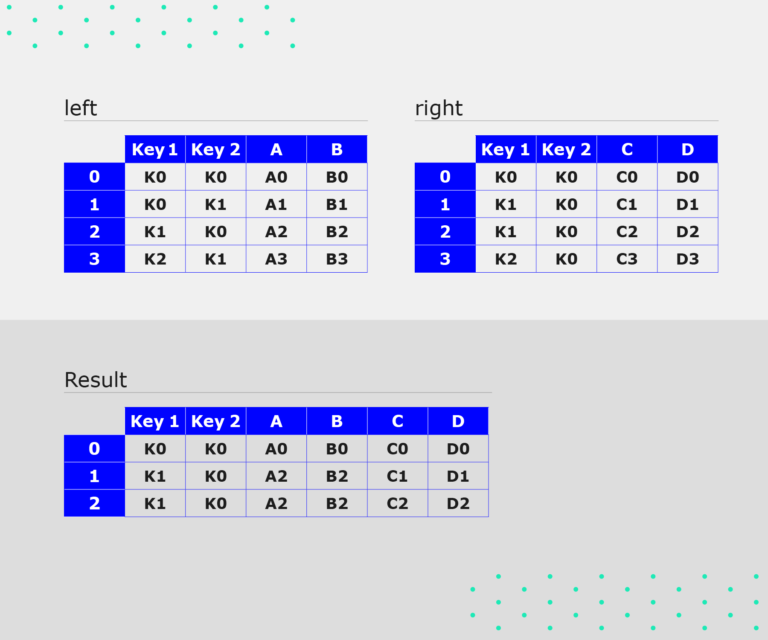Click to enlarge

Notice that both the left and right dataframes have the same key columns with the same values. If you do an inner join on the key column, you will get a Dataframe that has columns A and B from the left dataframe, and columns C and D from the right dataframe.

Try this code snippet in your Jupyter Notebook to see the process:

Code:

#Create DataFramesleft = pd.DataFrame({'key': ['K0', 'K1', 'K2', 'K3'], 'A': ['A0', 'A1', 'A2', 'A3'], 'B': ['B0', 'B1', 'B2', 'B3']})right = pd.DataFrame({'key': ['K0', 'K1', 'K2', 'K3'], 'C': ['C0', 'C1', 'C2', 'C3'], 'D': ['D0', 'D1', 'D2', 'D3']})#Merge DataFrame, perform Inner Joinpd.merge(left,right, on='key')

Output:Click to enlarge

## Example 2: Inner join with multi keys

Take a look at these dataframes: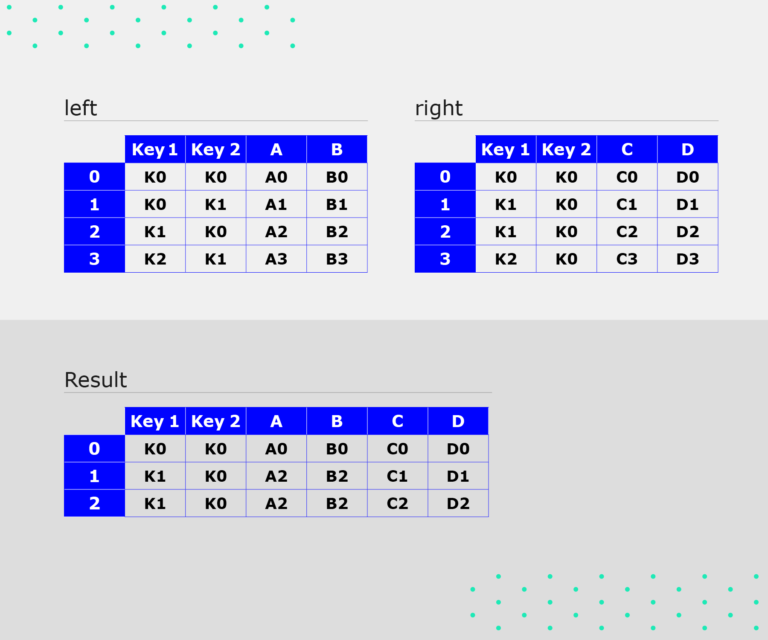Click to enlarge

Notice that:

• Both original dataframes have columns ‘key1’ and ‘key2’. If we perform an inner join on the two keys, the operation will use the combination of keys.

• The key pair (K0, K0) occurs in both original dataframes once, so it will be in the resulting dataframe once and the columns will be merged.

• The key pairs (K0, K1 and K2, K1) are not in the right-hand dataframe, so they won’t be in the resulting dataframe.

• Key pair (K1, K0), from the left-hand dataframe, occurs twice in the right-hand dataframe, so we will get two rows with this key in the resulting dataframe.

Try out the process with this code snippet:

Code:

left = pd.DataFrame({'key1': ['K0', 'K0', 'K1', 'K2'], 'key2': ['K0', 'K1', 'K0', 'K1'], 'A': ['A0', 'A1', 'A2', 'A3'], 'B': ['B0', 'B1', 'B2', 'B3']})right = pd.DataFrame({'key1': ['K0', 'K1', 'K1', 'K2'], 'key2': ['K0', 'K0', 'K0', 'K0'], 'C': ['C0', 'C1', 'C2', 'C3'], 'D': ['D0', 'D1', 'D2', 'D3']})result = pd.merge(left,right, on=['key1','key2'])result

Output:Click to enlarge

The how parameter determines how the join operation occurs (ie, which keys to include in the resulting table). If a key combination does not appear in either the left or right tables, the values in the joined table will be NA.

This table summarises join operations:

Merge method Equivalent SQL operation Description
outer FULL OUTER JOIN Use a union of keys from both frames

## Example 3: Left join

This diagram illustrates a left join: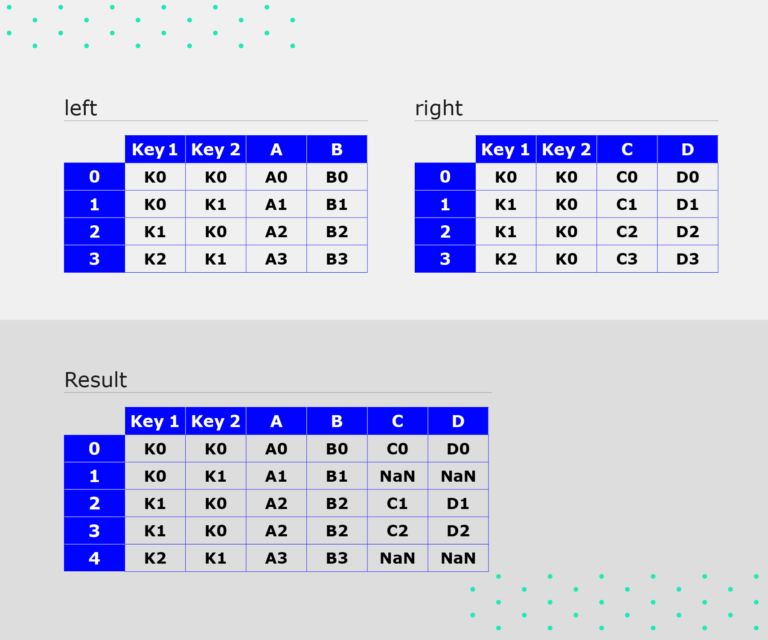Click to enlarge

Notice that:

• All the key pairs from the left-hand dataframe are available

• There are NA values in columns C and D if the key pair is not available in the right-hand dataframe

• The key pair (K1, K0) appears twice, because it appears twice in the right-hand dataframe (with different values in columns C and D).

Let’s use code to implement this join:

Code:

result=pd.merge(left,right, how='left', on=['key1','key2'])result

Output:Click to enlarge

## Example 4: Right join

Here is an example of a right join: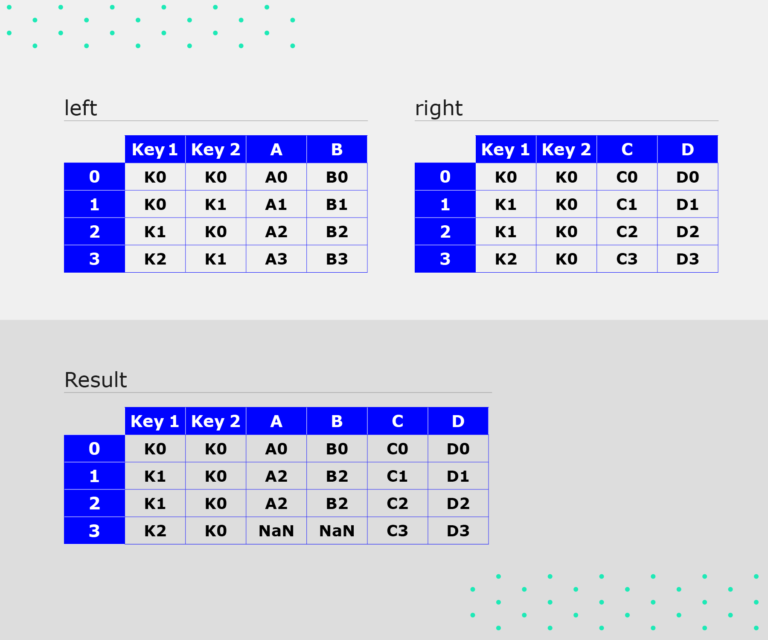Click to enlarge

As you can see, all the key pairs from the right-hand dataframe are available. Columns A and B have NA values where the key pair is not available in the right-hand dataframe.

Here is some code to implement the right join:

Code:

result=pd.merge(left,right, how='right', on=['key1','key2'])result

### What output did you get?

What output did you receive on the Jupyter notebook? Share in the Comments below.

## Example 5: Outer join

Look at this diagram: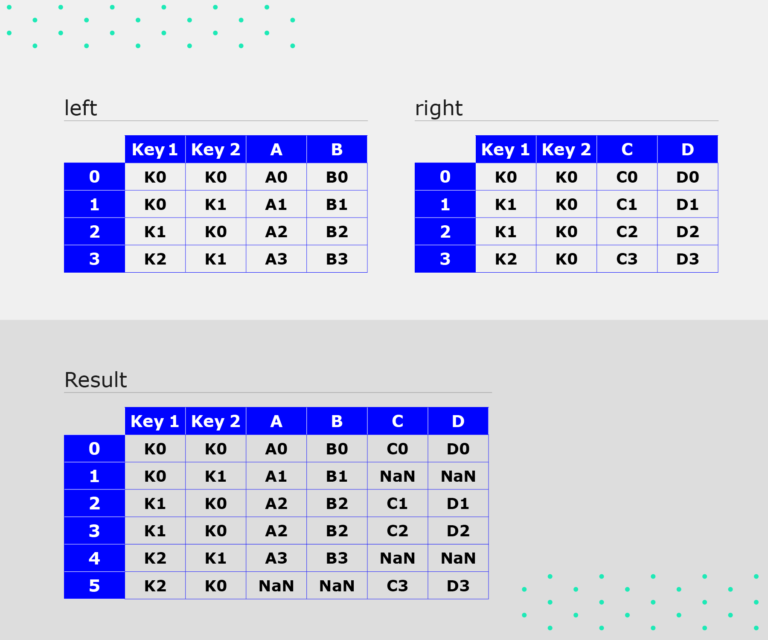Click to enlarge

Notice that all the key pairs from the left- as well as right-hand dataframe are present in the result. The NaN values in the columns correspond to key pairs that weren’t present in one of the original dataframes.

This code snippet demonstrates the operation:

Code:

result=pd.merge(left,right, how='outer', on=['key1','key2'])result

Output:Click to enlarge

You have already seen examples of inner joins in Examples 1 and 2, which is the default behaviour of merge(). There are many more possible variations of the merge() and join().

Go to: Merge, join, concatenate and compare 

#### Reference

1. Pandas User Guide: Merge, join, concatenate and compare. Pandas; [date unknown]. Available from: https://pandas.pydata.org/pandas-docs/stable/user_guide/merging.html